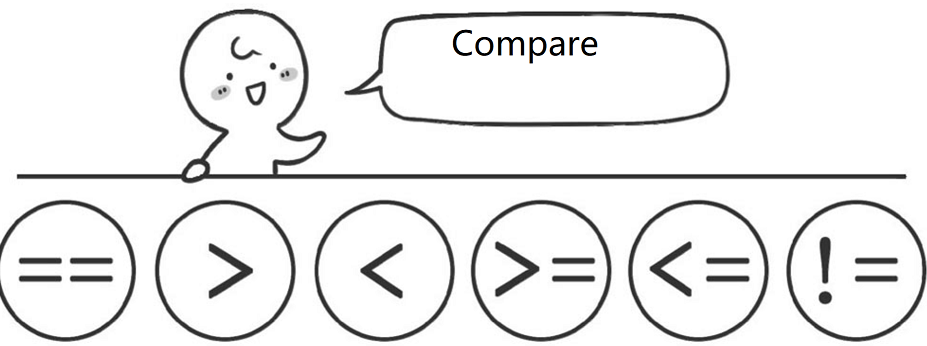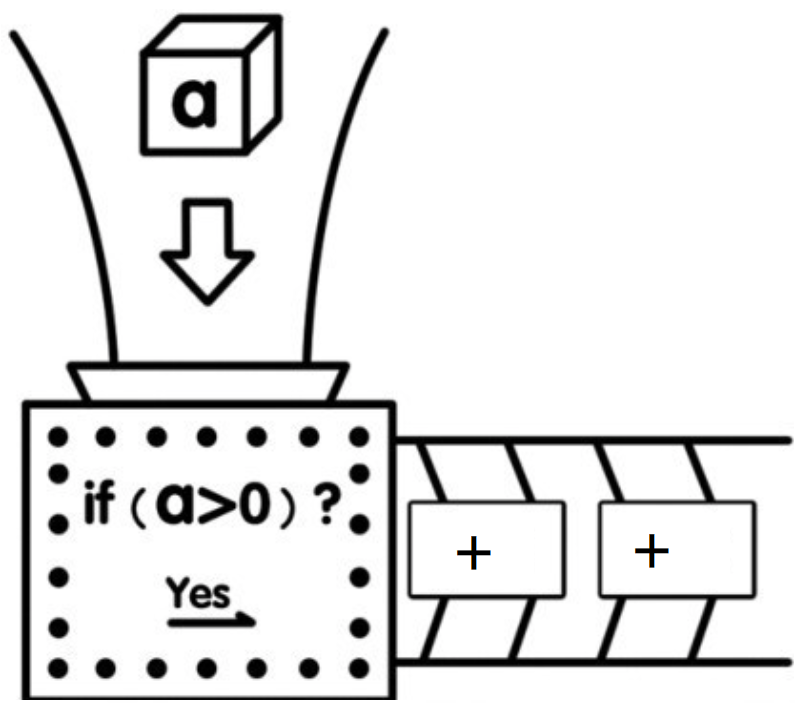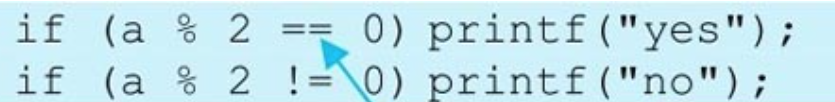# C Quick Tutorial -- Let the Computer Compare

Jump to Section

## Let the computer do “Compare”

Like us, computers can judge size. If you tell the computer that there are two numbers a and B, the computer can not only tell you the sum, difference, product and quotient of these two numbers, but also tell you who is bigger and who is smaller. Now let’s take a look at how computers judge who is big and who is small. == equal > greater than < less than >= Greater than or equal to <= Less than or equal to != Not equal to It should be noted that in the computer, one equals sign “=” indicates assignment, and two equal signs “= = =” indicates whether it is equal or not. Students must not make mistakes when writing code. An exclamation point plus an equal sign “! =” means “not equal to”. In addition, the computer only has the greater than or equal sign “> =” and the less than or equal to sign “< =”, without the equal greater than sign and equal to less than sign, namely “= >” and “= <”, which should be noted.

``````5>=4
7! =8
a<b
c==d
``````

The following is wrong: 4=<7 8=>3## Section 2 judge positive numbers

If you want the computer to judge whether an integer is a positive number, if it is, it will display yes, if not, what should be done?

The content in the box below is the “algorithm” for the computer to determine whether a number is positive.

First, the computer needs to have a small house (or variable) to store the number.

Then, you need to tell the computer what the number is?

Next, the computer needs to determine whether the number is positive.

Finally, the judgment result of the computer is output.

Algorithm is actually the way to solve the problem.Every time we encounter a problem, the first thing we need to think about is the algorithm to solve the problem, that is, the methods and steps to solve the problem. Step by step as above, and then each step of the algorithm is implemented by C language. Next, we use C language to implement the above algorithm. First, the computer needs to have a small house (or variable) to store the number.

Then, you need to tell the computer what this number is?

You can use scanf ("% d", & A); to read in a number and store it in small house a.

Next, the computer needs to determine whether the number is positive.

What can I do? It doesn’t matter. We’ll analyze it later.

Finally, the judgment result of the computer is output.

If it is a positive number, yes is displayed, using printf (“yes”);.

OK, now let’s focus on step 3, which is to determine whether the number stored in house a is positive.

Think about it. How do we judge whether a number is positive? From the definition of positive numbers, if a number is greater than 0, it is a positive number. OK, so does the computer. Ha ha. Next, try to use C language to realize. If is represented by if in C language. The code is as follows: if (a>0) { printf(“yes”); } When a > 0 is true, only one statement needs to be executed, and {} can be omitted, as follows: if (a>0) printf(“yes”); Note that if and only if the condition is true, only one statement needs to be executed to omit {}. Why do you say that? Don’t worry. You’ll know when you finish reading verse 7 of this chapter.

``````if (a>0)
printf("yes");
``````

A better way to write this statement is to use printf (“yes”); four blank spaces or one blank tab in front of it to indicate printf (“yes”); it is a part of if (a > 0), and printf (“yes”) is executed when a > 0 condition is true. if (a>0) printf(“yes”);

The complete code is as follows:

``````    #include <stdio.h>
#include <stdlib.h>
int main( )
{
int  a;
scanf("%d", &a);
if (a>0)  printf("yes");
system("pause");
return 0;
}
``````

## Section 3 even number judgment

Through the study of section 2 of this chapter, we know that computers judge by if statements. Now try to determine whether a number is even. First, write the algorithm.

(1) The computer needs a small house (or variable) to store the number. (2) You need to tell the computer what this number is? (3) The computer needs to determine whether the number is even. (4) The computer outputs the judgment results.

In step 3, you may have a little trouble. Let’s think, what is even? An even number is a number that can be divisible by 2, that is, if the remainder of a number divided by 2 is 0, then the number is even. Then we only need to judge whether the remainder of this number divided by 2 is 0, that is, if the remainder of a divided by 2 is equal to 0, then yes is output; if the remainder of a divided by 2 is not equal to 0, output No.

In C language, the operation symbol of remainder is “%”, so the C language code for judging whether a number is even is:The complete C language code is as follows:

``````    #include <stdio.h>
#include <stdlib.h>
int main( )
{
int  a;
scanf("%d", &a) ;
if (a%2==0)
{
printf("yes");
}
if (a%2! =0)
{
printf("no");
}
system("pause");
return 0;
}
``````

Well, it’s time to try.

[TO Be Continue]

## Report problems to us

Hi, if you find something need improved. Please feel free to contact us feedback. We will buy you a coffee if your advise is adopted. Thx.